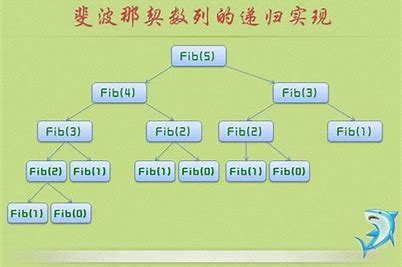# 算法：费波纳茨数列1 1 2 3 5 8 13 21## 方法一：循环实现

int fun(int n) {
int sum = 0, tmp1=1, tmp2 = 1;

if (n < 2)
return 1;

for(int i = 2; i <= n; i++) {
sum = tmp1 + tmp2;
tmp2 = tmp1;
tmp1 = sum;
}

return sum;
}


## 方法二：递归实现

int fun(int n) {
int sum = 0;

if (n < 2)
return 1;

for(int i = 2; i <= n; i++) {
sum = fun(i-2) + fun1(i-1);
}

return sum;
}©️2019 CSDN 皮肤主题: 编程工作室 设计师: CSDN官方博客